# Count Data And Overdispersion

## Overview

For count response variables, the glm framework has two options. The poisson family and the negative binomial family. In this vignette, we will consider both and learn when to use one or the other.

## Getting Familiar With The Poisson Family

First lets get an idea of how the poisson family looks. If lambda is near 1, the poisson family is right skewed. As lambda increases, the poisson family becomes more gaussian.

library(stats)
library(MASS)
library(dplyr, warn.conflicts= FALSE)
library(ggplot2)
library(GlmSimulatoR)

set.seed(1)

#lambda = 1
poisson <- rpois(n = 10000, lambda = 1)
poissonDF <- as.data.frame(x=poisson)

ggplot(poissonDF, aes(x=poisson)) +
geom_histogram(bins = 100)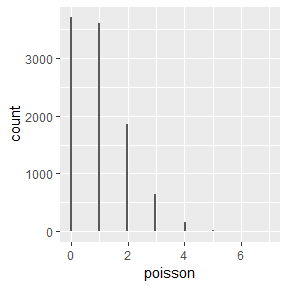#lambda = 5
poisson <- rpois(n = 10000, lambda = 5)
poissonDF <- as.data.frame(x=poisson)

ggplot(poissonDF, aes(x=poisson)) +
geom_histogram(bins = 100)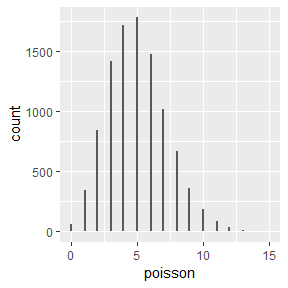#lambda = 10
poisson <- rpois(n = 10000, lambda = 10)
poissonDF <- as.data.frame(x=poisson)

ggplot(poissonDF, aes(x=poisson)) +
geom_histogram(bins = 100)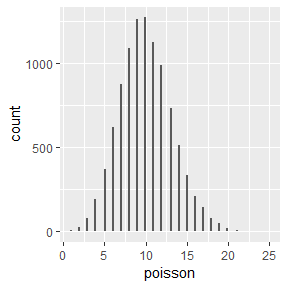## GLM Poisson

The below histogram looks similar to the above histograms. Also, the Maximum Likelihood Estimation in the GLM framework was able to estimate the slopes well.

set.seed(1)
simdata <- simulate_poisson(N = 10000, weights = c(.5, 1))

#Response looks similar to above histograms
ggplot(simdata, aes(x=Y)) +
geom_histogram(bins = 100)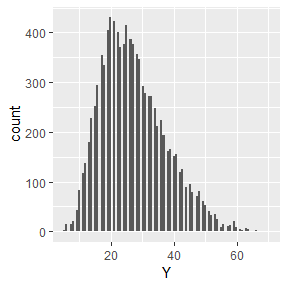glmPoisson <- glm(Y ~ X1 + X2, data = simdata, family = poisson(link = "log"))
summary(glmPoisson)
##
## Call:
## glm(formula = Y ~ X1 + X2, family = poisson(link = "log"), data = simdata)
##
## Deviance Residuals:
##     Min       1Q   Median       3Q      Max
## -3.7852  -0.7074  -0.0209   0.6427   3.4563
##
## Coefficients:
##             Estimate Std. Error z value Pr(>|z|)
## (Intercept) 0.996422   0.014963   66.59   <2e-16 ***
## X1          0.506335   0.006625   76.43   <2e-16 ***
## X2          0.996936   0.006816  146.26   <2e-16 ***
## ---
## Signif. codes:  0 '***' 0.001 '**' 0.01 '*' 0.05 '.' 0.1 ' ' 1
##
## (Dispersion parameter for poisson family taken to be 1)
##
##     Null deviance: 38086  on 9999  degrees of freedom
## Residual deviance: 10164  on 9997  degrees of freedom
## AIC: 60925
##
## Number of Fisher Scoring iterations: 4

## Poisson Mean Variance Relationship

One important characteristic of the poisson family is $$\mu$$ equals $$\sigma^2$$. We see the sample statistics are nearly equal for different values of lambda.

set.seed(1)

#lambda = 1
poisson <- rpois(n = 10000, lambda = 1)
mean(poisson)
##  1.0055
var(poisson)
##  1.00617
#lambda = 5
poisson <- rpois(n = 10000, lambda = 5)
mean(poisson)
##  4.9992
var(poisson)
##  4.999899
#lambda = 10
poisson <- rpois(n = 10000, lambda = 10)
mean(poisson)
##  10.04
var(poisson)
##  10.13221

## Poisson Vs Negative Binomial

For modeling, the main difference between poisson and the negative binomial is the extra parameter. The mean variance relationship is $$\sigma^2$$ equals $$\mu + \mu^2/\theta$$ for the negative binomial. Through $$\theta$$ many relationships are possible.

When the response variable is a count, but $$\mu$$ does not equal $$\sigma^2$$, the poisson distribution is not applicable. Overdispersion can be detected by dividing the residual deviance by the degrees of freedom. If this quotient is much greater than one, the negative binomial distribution should be used. There is no hard cut off of “much larger than one”, but a rule of thumb is 1.10 or greater is considered large.

## Getting Familiar With The Negative Binomial Family

When $$\theta$$ is large, $$\mu + \mu^2/\theta$$ is roughly equal to $$\mu$$ . Therefore the negative binomial will be similar to poisson distribution.

set.seed(1)

poisson <- rpois(n = 10000, lambda = 1)
poissonDF <- as.data.frame(x=poisson)

ggplot(poissonDF, aes(x=poisson)) +
geom_histogram(bins = 100)negBin <- rnegbin(n = 10000, mu = 1, theta = 1000)
negBinDF <- as.data.frame(x=negBin)

ggplot(negBinDF, aes(x=negBin)) +
geom_histogram(bins = 100)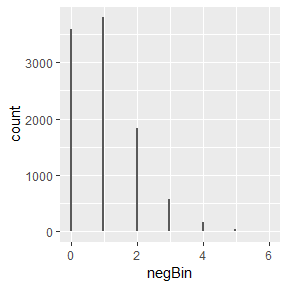If $$\theta$$ is small, $$\mu + \mu^2/\theta$$ does not approximately equal $$\mu$$. The negative binomial will look different than poisson distribution. Note the difference in scale on the x axis and y axis.

set.seed(1)

poisson <- rpois(n = 10000, lambda = 1)
poissonDF <- as.data.frame(x=poisson)

ggplot(poissonDF, aes(x=poisson)) +
geom_histogram(bins = 100)negBin <- rnegbin(n = 10000, mu = 1, theta = 1)
negBinDF <- as.data.frame(x=negBin)

ggplot(negBinDF, aes(x=negBin)) +
geom_histogram(bins = 100)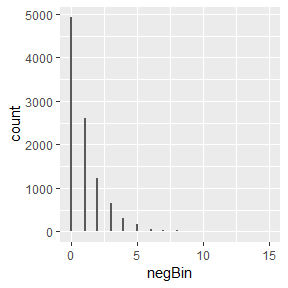## GLM Negative Binomial

Lets create data where the flexibility of the negative binomial is needed and compare the poisson estimates to the negative binomial estimates.

set.seed(1)
simdata <- simulate_negative_binomial(N = 10000, weights = c(.5, 1), ancillary = 5) #ancillary is theta.

#Response looks like a negative binomial distribution.
ggplot(simdata, aes(x=Y)) +
geom_histogram(bins = 200)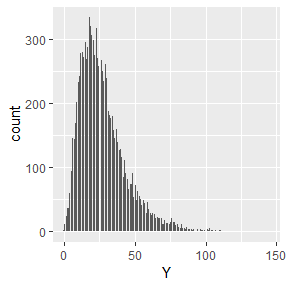glmPoisson <- glm(Y ~ X1 + X2, data = simdata, family = poisson(link = "log"))
glmNB <- glm.nb(Y ~ X1 + X2, data = simdata, link = "log")

summary(glmPoisson)
##
## Call:
## glm(formula = Y ~ X1 + X2, family = poisson(link = "log"), data = simdata)
##
## Deviance Residuals:
##     Min       1Q   Median       3Q      Max
## -7.8711  -1.9642  -0.3617   1.3337  11.0001
##
## Coefficients:
##             Estimate Std. Error z value Pr(>|z|)
## (Intercept) 0.995434   0.014925   66.70   <2e-16 ***
## X1          0.511899   0.006608   77.47   <2e-16 ***
## X2          0.995539   0.006797  146.46   <2e-16 ***
## ---
## Signif. codes:  0 '***' 0.001 '**' 0.01 '*' 0.05 '.' 0.1 ' ' 1
##
## (Dispersion parameter for poisson family taken to be 1)
##
##     Null deviance: 91470  on 9999  degrees of freedom
## Residual deviance: 63327  on 9997  degrees of freedom
## AIC: 113055
##
## Number of Fisher Scoring iterations: 4
summary(glmNB)
##
## Call:
## glm.nb(formula = Y ~ X1 + X2, data = simdata, link = "log", init.theta = 4.967762132)
##
## Deviance Residuals:
##     Min       1Q   Median       3Q      Max
## -4.0892  -0.8275  -0.1503   0.5161   3.7115
##
## Coefficients:
##             Estimate Std. Error z value Pr(>|z|)
## (Intercept)  0.98915    0.03658   27.04   <2e-16 ***
## X1           0.51132    0.01689   30.28   <2e-16 ***
## X2           1.00018    0.01707   58.60   <2e-16 ***
## ---
## Signif. codes:  0 '***' 0.001 '**' 0.01 '*' 0.05 '.' 0.1 ' ' 1
##
## (Dispersion parameter for Negative Binomial(4.9678) family taken to be 1)
##
##     Null deviance: 14739  on 9999  degrees of freedom
## Residual deviance: 10364  on 9997  degrees of freedom
## AIC: 77883
##
## Number of Fisher Scoring iterations: 1
##
##
##               Theta:  4.9678
##           Std. Err.:  0.0835
##
##  2 x log-likelihood:  -77874.7800

The estimated slopes are very similar. However, the standard errors are not. The fact $$\mu$$ does not equal $$\sigma^2$$ has caused a major under estimation of standard errors for the poisson GLM. For poisson models, it is important to look at residual deviance divided by the degrees of freedom. When this quotient is larger than 1, the negative binomial should be considered. In the above the quotient was 6.33 (63327 / 9997) for the poisson glm.